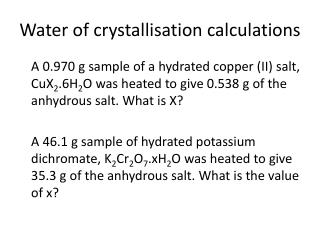DownloadDownload PresentationWater of crystallisation calculations

# Water of crystallisation calculations

Télécharger la présentation## Water of crystallisation calculations

- - - - - - - - - - - - - - - - - - - - - - - - - - - E N D - - - - - - - - - - - - - - - - - - - - - - - - - - -
##### Presentation Transcript

1. Water of crystallisation calculations A 0.970 g sample of a hydrated copper (II) salt, CuX2.6H2O was heated to give 0.538 g of the anhydrous salt. What is X? A 46.1 g sample of hydrated potassium dichromate, K2Cr2O7.xH2O was heated to give 35.3 g of the anhydrous salt. What is the value of x?

2. A 0.970 g sample of a hydrated copper (II) salt, CuX2.6H2O was heated to give 0.538 g of the anhydrous salt. What is X? Mass of H2O = mass of hydrated salt – mass of anhydrous salt = 0.970 – 0.538 = 0.432 g Moles of H2O = 0.432/18.0 = 0.024 moles Ratio CuX2 : H2O 1 : 6 So moles CuX2 = 0.024/6 = 0.004 Mr = mass/moles = 0.538/0.004 = 134.5 Mr of ‘X’ = (134.5-63.5)/2 = 35.5 X = Cl

3. A 46.1 g sample of hydrated potassium dichromate, K2Cr2O7.xH2O was heated to give 35.3 g of the anhydrous salt. What is the value of x? Moles of anhydrous salt = 35.3/294.2 = 0.120 moles Mass of H2O = 46.1 – 35.3 = 10.8 g Moles of H2O = 10.8/18.0 = 0.600 moles x = 0.600/0.120 = 5

4. The salt XSO4.5H2O was heated and 0.5 moles of water was lost. It left behind an anhydrous mass of 15.96g. What is X?

5. The salt XSO4.5H2O was heated and 0.5 moles of water was lost. It left behind an anhydrous mass of 15.96g. What is X? n XSO4 = 0.1 Mass XSO4 =15.96g Mr =mass/n Mr =15.96/0.1=159.6 Ar X =159.6-(32.1+64)=63.5 Therefore X = Cu